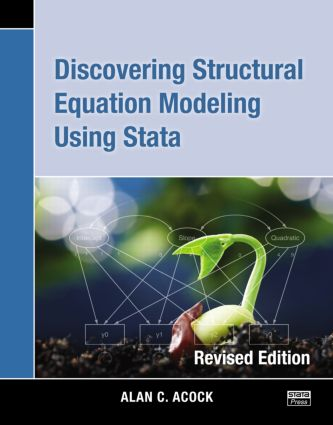Discovering Structural Equation Modeling Using Stata

Revised Edition, 1st Edition

Stata Press

306 pages

Paperback: 9781597181396
pub: 2013-09-10
x

FREE Standard Shipping!

Description

Discovering Structural Equation Modeling Using Stata, Revised Edition is devoted to Stata’s sem command and all it can do. Learn about its capabilities in the context of confirmatory factor analysis, path analysis, structural equation modeling, longitudinal models, and multiple-group analysis. Each model is presented along with the necessary Stata code, which is parsimonious, powerful, and can be modified to fit a wide variety of models. The datasets used are downloadable, offering a hands-on approach to learning.

A particularly exciting feature of Stata is the SEM Builder. This graphical interface for structural equation modeling allows you to draw publication-quality path diagrams and fit the models without writing any programming code. When you fit a model with the SEM Builder, Stata automatically generates the complete code that you can save for future use. Use of this unique tool is extensively covered in an appendix and brief examples appear throughout the text.

Introduction to confirmatory factor analysis

Introduction

The "do not even think about it" approach

The principal component factor analysis approach

Alpha reliability for our nine-item scale

Generating a factor score rather than a mean or summative score

Fitting a CFA model

Interpreting and presenting CFA results

Assessing goodness of fit

A two-factor model

Parceling

Extensions and what is next

Exercises

Using the SEM Builder to run a CFA

Using structural equation modeling for path models

Introduction

Path model terminology

A substantive example of a path model

Estimating a model with correlated residuals

Auxiliary variables

Testing equality of coefficients

A cross-lagged panel design

Moderation

Nonrecursive models

Exercises

Using the SEM Builder to run path models

Structural equation modeling

Introduction

The classic example of a structural equation model

Equality constraints

Programming constraints

Structural model with formative indicators

Exercises

Latent growth curves

Discovering growth curves

A simple growth curve model

Identifying a growth curve model

An example of a linear latent growth curve

How can we add time-invariant covariates to our model?

Explaining the random effects—time-varying covariates

Constraining variances of error terms to be equal (optional)

Exercises

Group comparisons

Interaction as a traditional approach to multiple-group comparisons

The range of applications of Stata’s multiple-group comparisons with sem

A measurement model application

Multiple-group path analysis

Multiple-group comparisons of structural equation models

Exercises

Epilogue—what now?

What is next?

The graphical user interface

Introduction

Menus for Windows, Unix, and Mac

Designing a structural equation model

Drawing an SEM model

Fitting a structural equation model

Postestimation commands

Clearing preferences and restoring the defaults

B Entering data from summary statistics